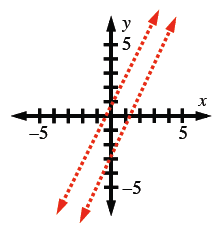### Home > AC > Chapter 4 > Lesson 4.2.4 > Problem4-98

4-98.

Graph the lines $y = 2x − 3$ and $y = 2x + 1$.1. Where do they intersect?

Do parallel lines ever intersect?

1. Solve this system using the Equal Values Method.

Set the equations equal to each other.

$y = y$
$2x − 3 = 2x + 1$

Subtract $2x$ from both sides. What happens?

1. Explain how your graph and algebraic solution relate to each other.

What do the two methods have in common?